# Ways of Expressing Concentration

## Concentration of A Solution

– The concentration of a solution is defined as : the amount of solute present in a given amount of solution.

– Concentration is generally expressed as the quantity of solute in a unit volume of solution.

A solution containing a relatively low concentration of solute is called Dilute solution.

– A solution of high concentration is called Concentrated solution.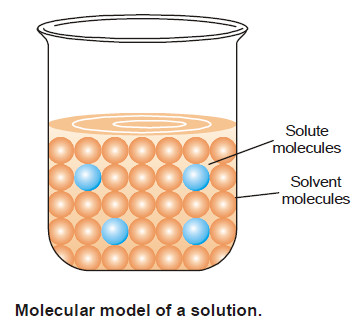## Ways of Expressing Concentration

– There are several ways of expressing concentration of a solution:

(a) Percent by weight

(b) Mole fraction

(c) Molarity

(d) Molality

(e) Normality

## (1) Percent by Weight

It is the weight of the solute as a per cent of the total weight of the solution.

That is,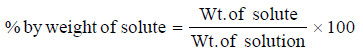For example, if a solution of HCl contains 36 per cent HCl by weight, it has 36 g of HCl for 100 g of solution.

### Solved problem on percent by Weight

What is the per cent by weight of NaCl if 1.75 g of NaCl is dissolved in 5.85 g of water?

SOLUTION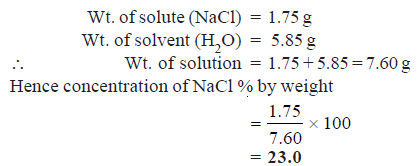## (2) Mole Fraction

– A simple solution is made of two substances : one is the solute and the other solvent.

– Mole fraction, X, of solute is defined as the ratio of the number of moles of solute and the total number of moles of solute and solvent.

Thus,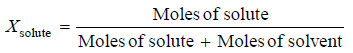If (n) represents moles of solute and N number of moles of solvent,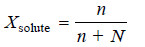Notice that mole fraction of solvent would be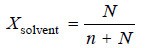Mole fraction is unitless and

Xsolute + Xsolvent = 1

### Solved problem on Mole fraction

Calculate the mole fraction of HCl in a solution of hydrochloric acid in water, containing 36 per cent HCl by weight

SOLUTION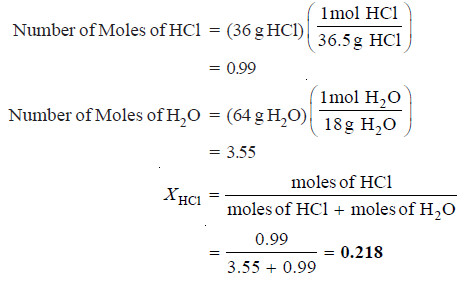## (3) Molarity

– In current practice, concentration is most often expressed as molarity.

– Molarity (symbol M) is defined as the number of moles of solute per litre of solution.

– If (n) is the number of moles of solute and (V) litres the volume of solution,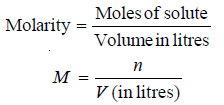– For one mole of solute dissolved in one litre of solution M = 1 i.e., molarity is one. Such a solution is called 1M (read “1 molar”).

– A solution containing two moles of solute in one litre is 2M (“two molar”); and so on.

– As evident from the previous equation, unit of molarity is mol litre–1.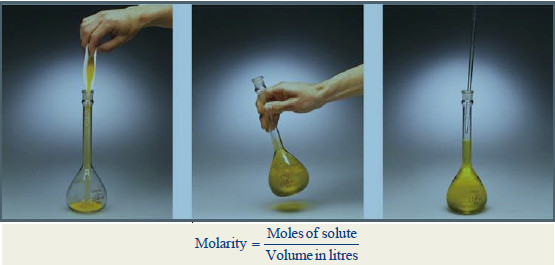### Calculation of Molarity

– Molarity of a solution can be calculated with the help of the expression (1) if moles of solute (n) and volume V (in litres) are known.

– When the amount of solute is given in grams and its molecular weight is MW, it can be converted to moles :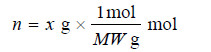Substituting in expression M = n / V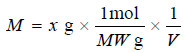– From the equation (M = n / V) can also be found the amount of solute in grams if molarity is given.

### Solved problem on Molarity

Problem(1): What is the molarity of a solution prepared by dissolving 75.5 g of pure KOH in 540 ml of solution.

SOLUTION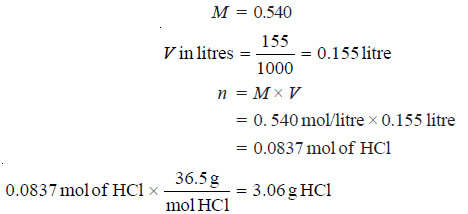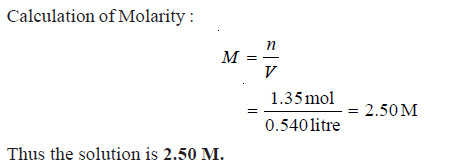Problem(2): What weight of HCl is present in 155 ml of a 0.540 M solution?

SOLUTION: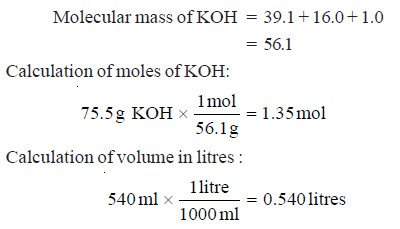## (4) Molality

Molality of a solution (symbol m) is defined as the number of moles of solute per kilogram of solvent: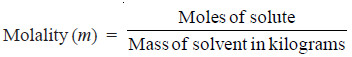–  A solution obtained by dissolving one mole of the solute in 1000 g of solvent is called one molal or 1m solution.

– Notice the difference between molality and molarity.

Molality is defined in terms of mass of solvent while molarity is defined in terms of volume of solution.

### Solved problem on Molality

What is the molality of a solution prepared by dissolving 5.0 g of toluene (C7H8) in 225 g of benzene (C6H6)?

SOLUTION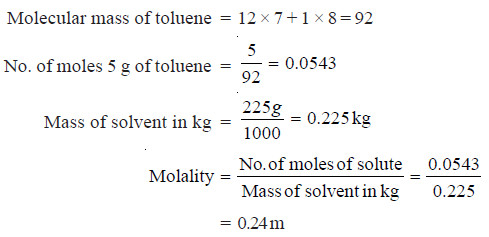## (5) Normality

Normality of a solution (symbol N) is defined as number of equivalents of solute per litre of the solution.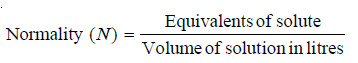Thus, if 40 g of NaOH (eq. wt. = 40) be dissolved in one litre of solution, normality of the solution is one and the solution is called 1N (one-normal). A solution containing 4.0 g of NaOH is 1/10 N or 0.1 N or decinormal.

### Solved problem on Normality

5 g of NaCl is dissolved in 1000 g of water. If the density of the resulting solution is 0.997 g per ml, calculate the molality, molarity, normality and mole fraction of the solute, assuming volume of the solution is equal to that of solvent.

SOLUTION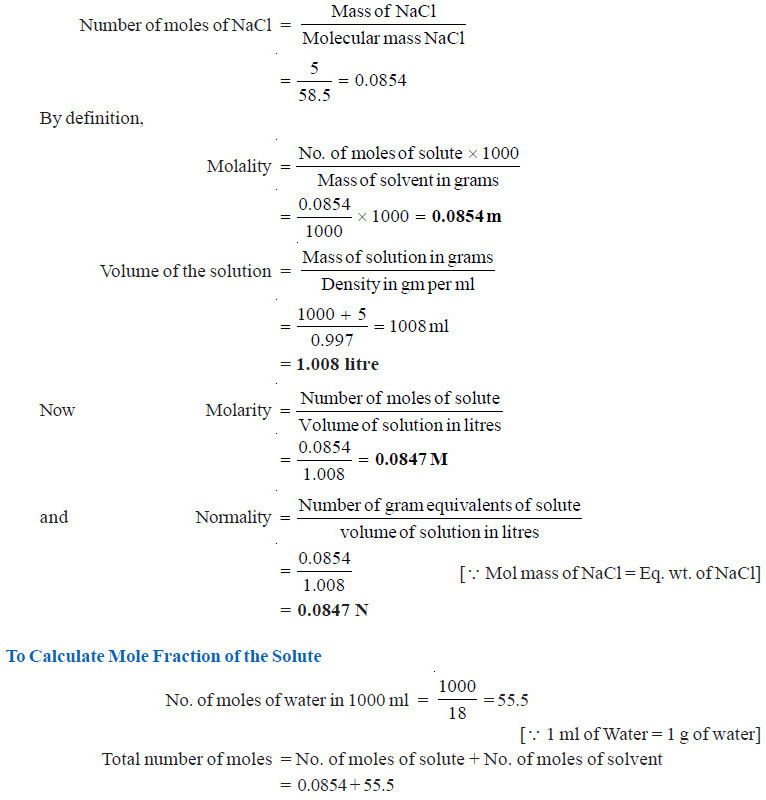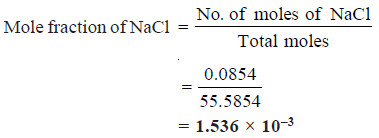### One comment

1.sees good